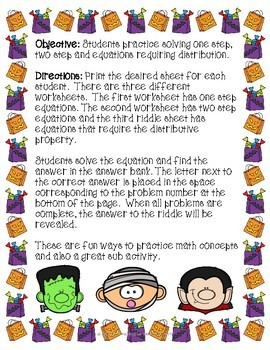DID YOU KNOW:
Seamlessly assign resources as digital activities

Learn how in 5 minutes with a tutorial resource. Try it Now

Learn More# Solving Equations Halloween Riddle7th - 10th
Subjects
Standards
Resource Type
Formats Included
• PDF
Pages
11 pages

### Description

Students practice solving one step, two step and multistep equations, find the answer and reveal the answer to a riddle about Halloween in this activity. There are three different worksheets, use as classwork, homework. Also makes a great sub plan

A great practice with a spooky twist.

Use the different worksheets after each individual lesson, or as a review before a test.

What is included:

☀3 different students "worksheets"

☀Teacher directions for use.

Total Pages
11 pages
Included
Teaching Duration
45 minutes
Report this Resource to TpT
Reported resources will be reviewed by our team. Report this resource to let us know if this resource violates TpT’s content guidelines.

### Standards

to see state-specific standards (only available in the US).
Solve linear equations and inequalities in one variable, including equations with coefficients represented by letters.
Solve simple rational and radical equations in one variable, and give examples showing how extraneous solutions may arise.
Solve linear equations with rational number coefficients, including equations whose solutions require expanding expressions using the distributive property and collecting like terms.
Give examples of linear equations in one variable with one solution, infinitely many solutions, or no solutions. Show which of these possibilities is the case by successively transforming the given equation into simpler forms, until an equivalent equation of the form 𝘹 = 𝘢, 𝘢 = 𝘢, or 𝘢 = 𝘣 results (where 𝘢 and 𝘣 are different numbers).
Solve linear equations in one variable.